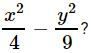Courses

# Test: Factorisation- 3

## 20 Questions MCQ Test Mathematics (Maths) Class 8 | Test: Factorisation- 3

Description
This mock test of Test: Factorisation- 3 for Class 8 helps you for every Class 8 entrance exam. This contains 20 Multiple Choice Questions for Class 8 Test: Factorisation- 3 (mcq) to study with solutions a complete question bank. The solved questions answers in this Test: Factorisation- 3 quiz give you a good mix of easy questions and tough questions. Class 8 students definitely take this Test: Factorisation- 3 exercise for a better result in the exam. You can find other Test: Factorisation- 3 extra questions, long questions & short questions for Class 8 on EduRev as well by searching above.
QUESTION: 1

### What are the factors of ax+by+bx+az+ay+bz?

Solution:

ax+by+bx+az+ay+bz, rearranging we get ax+ay+az+bx+by+bz
a(x+y+z)+b(x+y+z)=(a+b)(x+y+z). Hence the factors are (a+b), (x+y+z)

QUESTION: 2

### Which of the following is one of the factors of x4+4?

Solution:

x⁴ + 4

= (x² + 2)² - 4x²

= (x² + 2)² - (2x)²

a² - b² = (a + b)(a - b)

= (x² + 2 + 2x)(x² + 2 - 2x)

QUESTION: 3

### What are the factors of x4+2x2+9?

Solution:
QUESTION: 4

For x2+2x+5 to be a factor of x4+ px2+q, what must the respective values of p and q be?

Solution:

Let the other factor be x2+ax+b. We have (x2+2x+5)(x2+ax+b)
= x4+px2+q
x4(2+a)x3(2a+b+5)x2(5a+2b)x+5b = x4+px2+q
Comparing the coefficients of corresponding terms, we get 2a+b+5 = p  ......(1)
5b = q   ......(2)
2+a = 0 ⇒ a =−2
5a+2b = 0 ⇒ b = 5
∴ p = 2a+b+5 = 2(−2)+5+5 = 6
q = 5b = 5(5) = 25

QUESTION: 5

What are the factors of x2+xy−2xz−2yz?

Solution:
QUESTION: 6

Amrit and Pankaj expanded (x−5)2. Amrit's answer is x2−25 and Pankaj's answer is x2−10x+25. Which of the following statements is correct?

Solution:

(x−5)2 = x2−10x+25 using

(a−b)2 = a2−2ab+b2. So, Pankaj's answer is correct.

QUESTION: 7

Find the quotient when 5a2b2c2 is divided by 15abc.

Solution:
QUESTION: 8

Which of the following statements is correct?

Solution:

(a−4)(a−2) = a2−4a−2a+8 = a2−6a+8 So, the statement in option (A) is correct.

QUESTION: 9

What are the factors of x4+y4+x2y2?

Solution:

x4+y4+x2y2 =(x4+y4+2x2y2)−x2y2 =(x2+y2)2−(xy)2
=(x2+y2+xy)(x2+y2−xy)

QUESTION: 10

Choose the factors of 15x2−26x+8 from the following.

Solution:

15x2−26x+8 =15x2−20x−6x+8 =5x(3x−4)−2(3x−4) =(3x−4)(5x−2)

QUESTION: 11

How many factors does (x9−x) have?

Solution:
QUESTION: 12

Which of the following are the factors ofSolution:
QUESTION: 13

What is the coefficient of 'a' when 9a2+18a is divided by (a+2)?

Solution:
QUESTION: 14

From the following, which are the factors of a2+b−ab−a?

Solution:
QUESTION: 15

The expression (p2+7p+10) is factorized and then divided by (p+5). What is the quotient?

Solution:
QUESTION: 16

Which is the correct statement in the following?

Solution:

n(3n+2) = 3n2+2n

QUESTION: 17

If (x2+3x+5)(x2−3x+5) = m2−n2, what is the value of m?

Solution:

(x2+3x+5)(x2−3x+5) = (x2+5+3x)(x2+5−3x) = (x2+5)2−(3x)= m2−n2
∴ m = x2+5

QUESTION: 18

Divide 6p5+18p4−3p2 by 3p2.

Solution:
QUESTION: 19

Find the factors of b2−7b+12.

Solution:

b- 7b+12=b2-3b-4b+12=b(b-3)-4(b-3)=(b-3)(b-4)

QUESTION: 20

Find the factors of6 mn−4n+6−9m.

Solution: